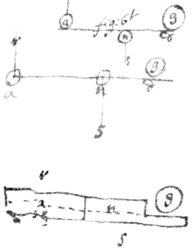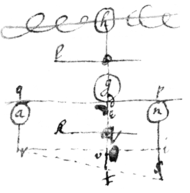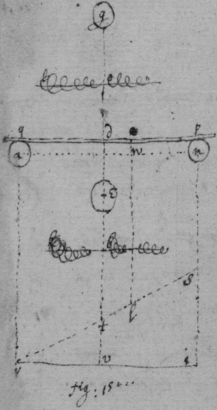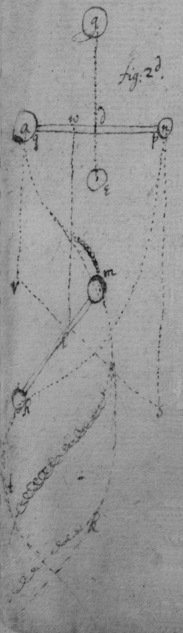Catalogue Entry: NATP00220

Newton's Waste Book (Part 1)

Source: MS Add. 4004, ff. {cover}-15r, Cambridge University Library, Cambridge, UK

 {Se}{p}t 1664.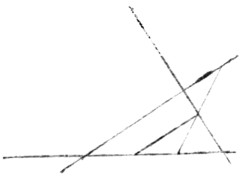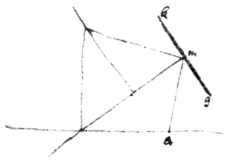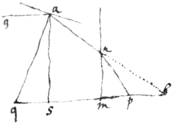{illeg} $2x+\frac{1}{3}xx={gd}^{2}$. $xx+4x+1={ag}^{2}$.

 $\begin{array}{l}\underset{_}{36}\hfill \\ 2025\\ \underset{_}{162\phantom{0}}\\ \phantom{0}405\end{array}$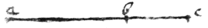$\begin{array}{l}\underset{_}{29376}\\ \underset{_}{162}\\ \underset{_}{29376}\\ \phantom{0}\underset{_}{7776}\\ \phantom{0}\underset{_}{729\phantom{0}}\\ \phantom{00}\underset{_}{486}\end{array}$ $\underset{\text{'}}{a}____________\underset{\text{'}}{b}_____\underset{\text{'}}{c}$ $\begin{array}{l}\underset{_}{32076×25dd}=801900dd\\ 160380\\ \underset{_}{64152}\end{array}$ $\begin{array}{l}729\\ \phantom{0}7290\end{array}$ $\begin{array}{l}134\\ \phantom{0}537\\ \phantom{0}\underset{_}{486}\\ \phantom{00}5184\\ \phantom{00}486\\ \phantom{000}324\end{array}$ $\begin{array}{l}\phantom{0}1296\\ \phantom{0}648\\ 962\end{array}\right\}=\begin{array}{c}\underset{_}{104976×9}\\ 944784\end{array}$ $\begin{array}{c}\phantom{0}900\\ \phantom{0}36\\ 1800\end{array}$ $\begin{array}{l}\phantom{0}405\\ \underset{_}{162\phantom{0}}\\ \underset{_}{2025×25dd}=50625\\ 10125\\ 4050\end{array}$ $\begin{array}{l}Q:\underset{_}{324}=104976\\ \phantom{0}1296\\ \phantom{0}648\\ 972\end{array}$ $\begin{array}{l}\phantom{0}216\\ \underset{_}{106}\\ \phantom{000}6\end{array}$ $\begin{array}{cc}36& \\ & \begin{array}{r}\phantom{0}216\\ \underset{_}{106}\phantom{0}\end{array}\\ & 1276\\ & 11484\end{array}$ $\begin{array}{rr}9×25×& 36\\ 3& 24\\ 16& 20\\ \underset{_}{64}& \underset{_}{8}\hfill \\ 81& 00\\ \underset{_}{18}& \underset{_}{00}\hfill \\ 99\end{array}$$\begin{array}{r}36×324\\ \phantom{0}1944\\ \underset{_}{\phantom{0}972\phantom{0}}\\ 11664\end{array}$ $x+\frac{r}{q}xx=9$. $\frac{r}{2}+\frac{rxq}{q}=2$ {illeg}$x=2x-\frac{rx}{2}$ . $\frac{rx}{2}+2x=9$.$x=3$. $rx=6$ $r=2$ $rx+4x=18$ $-\frac{qr}{2}+2q=rx$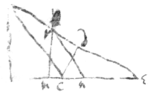$2q-\frac{qr}{2}+4x=18$ $\frac{qr}{2}+\frac{72}{r+4}-18=0$

 $cc=52c-117$. $\begin{array}{r}c=2 6-\sqrt{156}\phantom{\left(0}\\ \underset{_}{520}\phantom{\left(0}\\ 559\left(2\\ 4\\ 1\phantom{00\left(0}\end{array}$ $cc=80c-180$

 $\begin{array}{c}& \begin{array}{l}1600\\ 14\\ 1420\left(3\\ \phantom{00}20\end{array}\\ \phantom{0}\\ \begin{array}{ccc}13& .& \\ 14& .& 192\\ 15& .& 225\\ 16& .& 5\\ 17& .& 289\\ 18& .& 324\\ 19& .& 361\\ 20& .& 400\end{array}\end{array}$

 {illeg}${x}^{3}+{y}^{3}-axy=0$. $v=\frac{3xxy-ayy}{ax-3yy}$ $x+\frac{3xx{y}^{2}-ayyz}{\sqrt{\begin{array}{c}9{x}^{4}yy-6axx{y}^{3}\\ +aa{y}^{4}+aaxxyy\\ -6{a}^{3}xyy+9{a}^{4}{y}^{4}\\ \end{array}}}$ for x. $x+\frac{3zxx-azy}{\sqrt{\begin{array}{c}9{x}^{4}-6ayxx+10aayy\\ +aaxx-6{a}^{3}x\end{array}}}$ for x . $y\frac{+3yyz-azx}{\sqrt{\begin{array}{c}9{x}^{4}-6ay{x}^{2}+10{a}^{2}{y}^{2}\\ +aa{x}^{2}-6{a}^{3}x\end{array}}}$ for y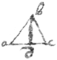$ab=a=2ad$ $ad=\frac{\sqrt{3aa}}{4}${illeg}$=x$. $ce=y$. $af=q$. {illeg}$x-xx=yy$. $ed=z$. $ed=s$. {illeg}$c=q-x$. ${eb}^{2}=qq$. $eb=q$. {illeg}$:2qx-xx:zz:$ ${eh}^{2}$. $eh=\sqrt{\frac{2qxzz-xxzz}{qq}}$ {illeg}= $\sqrt{2qx-xx}-\frac{z}{q}\sqrt{\phantom{00000}xx}$ {illeg} $=2qx-xx+\frac{2zzqx-\phantom{000}}{qq}$ {illeg}$-4zx+\frac{2zxx}{q}$ {illeg}$=\frac{qz-xz}{q}$ {illeg}$-\frac{zx}{q}$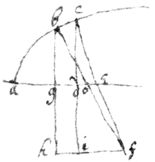$\begin{array}{cccc}& dxqy& +& 2fxyy\\ -& 2pfxy& -& byx\\ -& 2ayyx& -& \frac{dexy-ddyyy}{f}\end{array}$

 {illeg} $\begin{array}{ccccc}xy& +& ay& =& 0\\ ax& +& yy\end{array}$$ed=v$. $cd=x$. $ac=y$. $ab=s$. {illeg}$=z$. $eg=\xi$. $0=yy-2xy+ax-{x}^{2}$ $y=$ $\phantom{\rule{0.5em}{0ex}}\stackrel{\cup }{O}\phantom{\rule{0.5em}{0ex}}\sqrt{2xx-ax}=ac$ $yy=\begin{array}{c}2xx\\ -ax\end{array}\phantom{\rule{0.5em}{0ex}}\stackrel{\cup }{O}\phantom{\rule{0.5em}{0ex}}2\sqrt{2{x}^{4}-a{x}^{3}}$. ${eg}^{2}=\begin{array}{c}3{x}^{2}-60x-ax+ao\\ \phantom{\rule{0.5em}{0ex}}\stackrel{\cup }{O}\phantom{\rule{0.5em}{0ex}}\sqrt{2{x}^{4}-a{x}^{3}}\end{array}$. ${eg}^{2}=\begin{array}{c}3{z}^{2}\\ -az\end{array}\phantom{\rule{0.5em}{0ex}}\stackrel{\cup }{O}\phantom{\rule{0.5em}{0ex}}\sqrt{2{z}^{4}-a{z}^{3}}$ $yy-2xy+ax-xx=0$ $\frac{-2yy+ay-2xy}{-2y+2x}+x=v$ $x+y+\frac{ay}{2x-2y}=v$ $8{x}^{4}-4a{x}^{3}+4a{z}^{3}-8{z}^{4}$ $\begin{array}{r}{x}^{3}+4xxz+4xzz+4{z}^{3}\\ -2xxa-2axz-2a{z}^{2}\end{array}$ $\begin{array}{ccc}\sqrt{2xx-ax}& -& 2z\sqrt{2zz-az}\\ & +& 2z\sqrt{2xx-ax}\end{array}$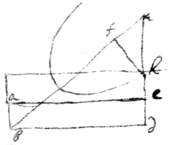$ab=a$. $bd:be\colon\colon b:c$. $\frac{cx}{b}=be$ . $\frac{cϩ}{b}=eh$. ${fe}^{2}==\frac{ccϩϩ-bbϩϩ}{bb}$ $fe=\frac{cx-bz}{b}=\frac{ϩ\sqrt{cc-bb}}{b}$ $\frac{bz+ϩ\sqrt{cc-bb}}{c}=x$ $ed=\frac{x\sqrt{cc-bb}}{b}$ $ed=\frac{ϩcc-ϩbb+bz\sqrt{cc-bb}}{bc}$ $eh=\frac{-ϩb+z\sqrt{cc-bb}-ca}{b}$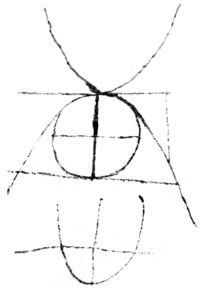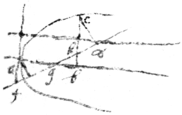$ϩ\sqrt{ec-dd}=ex$ .

 {illeg}$\begin{array}{c}\phantom{0}\\ \phantom{0}\end{array}+4{d}^{3}{\varrho }^{3}ϩ\sqrt{ee-dd}+$

 {illeg}$\begin{array}{c}\phantom{0}\\ \phantom{0}\\ \phantom{0}\end{array}{\varrho }^{5}+5{d}^{4}{\varrho }^{4}ϩ\sqrt{ee-dd}$

 {illeg}$\begin{array}{c}\phantom{0}\\ \phantom{0}\end{array}-dϩ+\varrho \sqrt{ee-dd}=ey$.

 $\varrho \phantom{\rule{1em}{0ex}}\begin{array}{l}-2dd\varrho ϩ\\ +ee\varrho ϩ\end{array}\phantom{\rule{1em}{0ex}}\begin{array}{l}\phantom{0}\\ +ceϩ\sqrt{ee-dd}\\ \end{array}=eexy$.

 $\begin{array}{c}d{ϩ}^{3}\\ {ϩ}^{3}\end{array}\phantom{\rule{1em}{0ex}}+2ce\varrho ϩ\sqrt{ee-dd}\phantom{\rule{1em}{0ex}}\begin{array}{ll}+& 2dee\varrho \varrho ϩ\\ -& 3{d}^{3}\varrho \varrho ϩ\end{array}\right\}={e}^{3}xxy$

 {illeg} $x+ax+bb=0$ . {illeg} $+\frac{aa}{0}+bb$

 $ac=d$. {illeg}$=a$. $ad=b$. $ed=c$. $\frac{aa}{b}=ab$. $ae=e$ {$\frac{ae}{b}=eb$.} $bc=\frac{db-aa}{b}$ {illeg} ${c}^{2}=$ $cc$ . $\frac{ddbb-2aabd+{a}^{4}+aacc}{bb}=ee$.{illeg}$d=a$. $pg=b$. $pd=g$ $\frac{ea}{d}=dh$ . $\frac{fa}{d}=gh$ . $h=\frac{bd-fa}{d}$ . {illeg} $bdd-2bdfa+aaff+eeaa=bbgg$ {illeg} $\begin{array}{c}aee\\ aadd\end{array}=2bda\sqrt{ee-dd}+bbgg-bbdd$ . {illeg} $a=\frac{bd\sqrt{ee-dd}}{2ee-dd}\phantom{\rule{0.5em}{0ex}}\stackrel{\cup }{O}\phantom{\rule{0.5em}{0ex}}\sqrt{\frac{-bbddee+2bbggee-bbggdd}{4{e}^{4}-4eedd+{d}^{4}}}$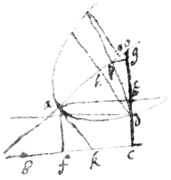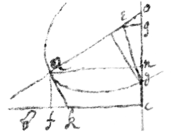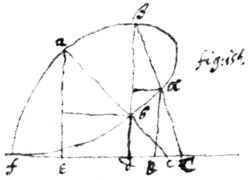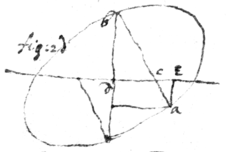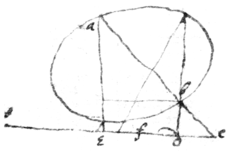September 1664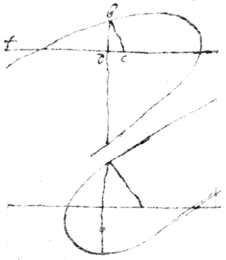$\frac{d\varrho +sϩ}{e}=x$. $\frac{t\varrho -vϩ+ce}{e}=$ y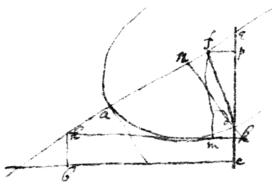$dg-eg-e\sqrt{dd-gg}+d\sqrt{dd-gg}=0$. $g=\sqrt{dd-gg}$. $dd=2gg$ or $d=e$ $ag-2cg-2c\sqrt{dd-gg}=0$ $\frac{ag}{2g+2\sqrt{dd-gg}}=c$. ${g}^{3}=-dd+gg\sqrt{dd-gg}$. $2gg=dd$. $-3ccdd\sqrt{dd-gg}=0=c$. $3ddg=3ee\sqrt{dd-gg}$ $d=e$. $-aegd$ $\begin{array}{l}-6cde\sqrt{dd-gg}=adeg=0\\ +add\hfill \end{array}$ $-3ddcc\sqrt{dd-gg}-addcg=0$ $9ccdd-9ccgg=aagg$ . ${d}^{4}gg=$ {${t}^{4}-$}$gg{e}^{4}$ $\begin{array}{llllll}& 36ccddee& -& 36ccggee& =& aaggee\\ +& aa{d}^{4}& -& aaddgg\\ -& 2cea{d}^{3}\end{array}$ $-r\sqrt{rx}-6x\sqrt{rx}$ $\begin{array}{ccccccc}3rx& +& 8xx& +& \frac{16{x}^{3}}{r}& +& \frac{rr}{4}\\ & +& 4xx\end{array}$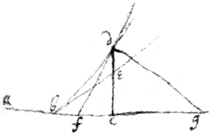Ian 20th 1664.Noe motion is lost in reflection. For the circular motion being made by continuall reflection would decay.Def 3d

 {illeg}s Axiome 4th.p Axiom 4th.Of the seperation of body{s} after reflection

 r axiome 3dThe center of motions determinacon & velocity

 x axiom 14Of endeavor from the center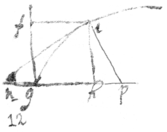let this follow the 5t axiomWhat force is required to beget or destroy equall velocity in unequall bodysWhat resistance in bodys

 What force Indeavor & Pression is

 What force or Motion is in equivelox bodysWhat velocity acquired or lost in equall bodys by unequall forces

 What motion in bodysA generall Theorem of the proportion of velocity & motion of given body moving ☞ through given spaces in given times.

 What force required to beget or destroy unequall celerity in equall bodys

 Of hindering and helping motion

 What celerity acquired or lost by equall forces in unequall bodysWhat velocity & motion gotten or lost by unequall forces in unequall bodys ☞ A Generall Theorem.

 Of the {illeg} force in reflected bodys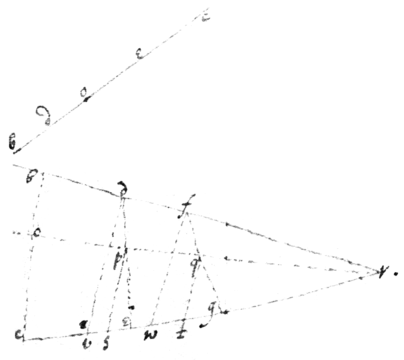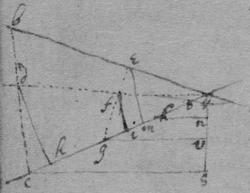Two bodys being uniformely moved in the same plaine their center of motion which describe a streight line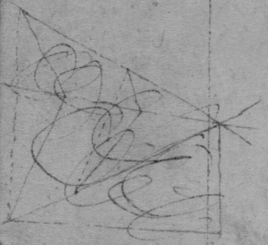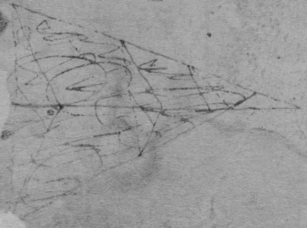{illeg} the {illeg} {as divers plaines}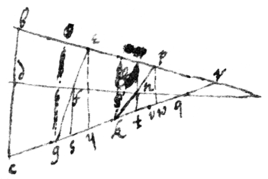of the velocity of the center of motion 14

 The 28th & 30th proposition done otherwise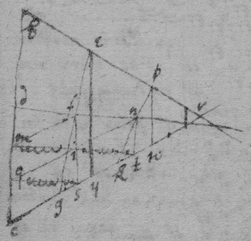Or thusThe {illeg} of motion is {illeg} before after {illeg}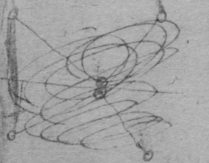The center of motion in finite bodys hath the same velocity before & after reflectionThis ought to be proved by the 34th & 35t, & the 36t by this concerning the impresse of $\left(g\right)$ on $\left(qdp\right)$Of the Advantage of force in divers positions to some center.